Custom math worksheets at your fingertips# Math worksheet template "Angles1"

This is not a ready-to-download math worksheet. It is a template, that is a preconfgured list of problems with parameters, that you can use to create your own math worksheet from. You can then change the resulting worksheet if some of the parameters need any tweaking. To use this template and create your own custom worksheet, you have to register first so you can edit worksheets. You can register and create an account in a minute - there is a free trial available. Register here, and you can use this template. There is no charge for joining and as a new member, you will get a free amount of starting credits. After registering, please choose the tab "My worksheets", then select "View templates".

## Title

Angles and angle types

## Description

Measure and draw angles, identify types of angles

## Elaboration

The problems on this worksheet deal with measuring and drawing angles, and correctly determining the angle type. For the first task, angles up to 90 degrees are to be measured. The next two tasks require the drawing of angles, first up to 90 degrees, then up to 180 degrees. In the last two exercises, angle types are to be determined, first for simpler angles excluding null and full angles, then for general angles.

## Preview

This is what the worksheet will look like. On the left hand side, the problem type is shown with its quickname. On the right, you will see a preview of the problem. This is just a sample, as dw-math will generate a new one when you load the template. Click on the underlined problem name to jump to the problem's detail page. You can download preview worksheets and solution sheets for this problem there. Note that all problem numbers are show as one here, in the worksheet they will be numbered sequentially. The worksheet's header and footer are not shown here.

 Angle size measurementMeasure the size of a given angleQuickname: 4788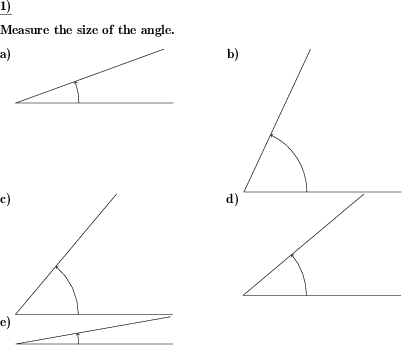Angle to be drawnAn angle of a given size has to be drawn.Quickname: 7461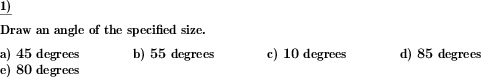Angle to be drawnAn angle of a given size has to be drawn.Quickname: 7461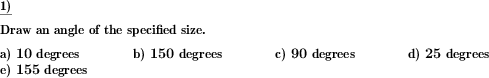Angle typesFor a given angle, the correct type has to be marked.Quickname: 6921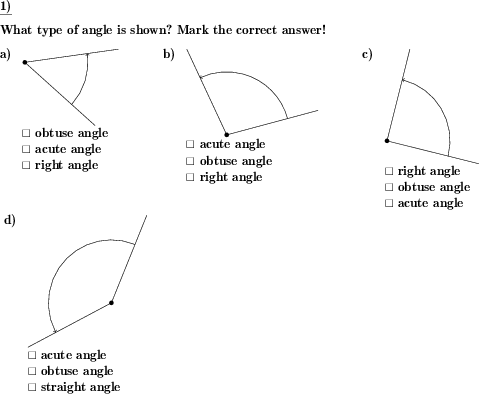Angle typesFor a given angle, the correct type has to be marked.Quickname: 6921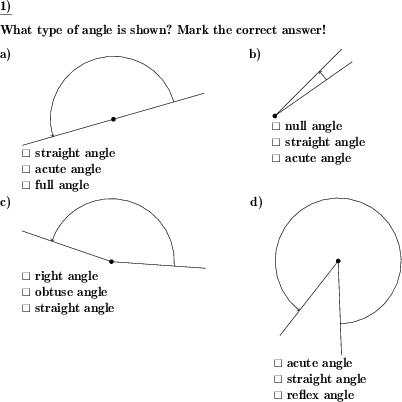These informational pages with samples describe math problems that can be combined on custom math worksheets with solutions for home and K-12 school use.